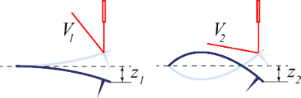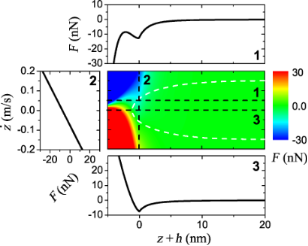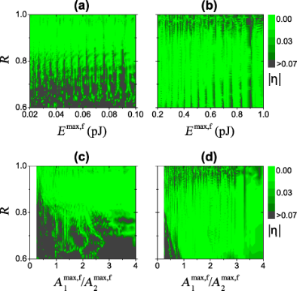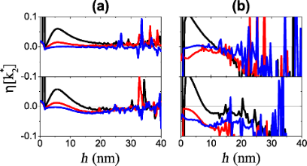# Dynamic calibration of higher eigenmode parameters of a cantilever in atomic force microscopy by using tip–surface interactions

1Nanostructure Physics, KTH Royal Institute of Technology, Roslagstullsbacken 21, SE-106 91 Stockholm, Sweden
2Nordita, KTH Royal Institute of Technology and Stockholm University, Roslagstullsbacken 23, SE-106 91 Stockholm, Sweden
3Theoretical Division, Los Alamos National Laboratory, Los Alamos, NM 87545, USA

1. Corresponding author email

Guest Editors: T. Glatzel and T. Schimmel
Beilstein J. Nanotechnol. 2014, 5, 1899–1904. https://doi.org/10.3762/bjnano.5.200
Received 10 Jun 2014, Accepted 01 Oct 2014, Published 29 Oct 2014

• Full Research Paper

## Abstract

We present a theoretical framework for the dynamic calibration of the higher eigenmode parameters (stiffness and optical lever inverse responsivity) of a cantilever. The method is based on the tip–surface force reconstruction technique and does not require any prior knowledge of the eigenmode shape or the particular form of the tip–surface interaction. The calibration method proposed requires a single-point force measurement by using a multimodal drive and its accuracy is independent of the unknown physical amplitude of a higher eigenmode.

Keywords: atomic force microscopy; calibration; multimodal AFM; multifrequency AFM

## Introduction

Atomic force microscopy  (AFM) is one of the primary methods of surface analysis with resolution at the nanometer scale. In a conventional AFM an object is scanned by using a microcantilever with a sharp tip at the free end. Measuring cantilever deflections allows not only for the reconstruction of the surface topography but also provides insight into various material properties [2,3]. If deflection is measured near one of the cantilevers resonance frequencies, an enhanced force sensitivity is achieved due to multiplication by the sharply peaked cantilever transfer function. Measurement of response at multiple eigenmodes can provide additional information about the tip–surface interactions [4-11].

The optical detection system  common to most AFM systems leverages a laser beam reflected from the cantilever, measuring the slope rather than its vertical deflection. This underlying principle leads to the measured voltage at the detector being dependent on the geometric shape of the excited eigenmode (Figure 1). While determination of the stiffness and optical lever inverse resposivity (inverse magnitude of the response function of the optical lever [m/V], also known as “inverse optical lever sensitivity”) of the first flexural eigenmode can be performed with high accuracy using a few well-developed techniques [13-21], calibration of the higher eigenmode parameters is still a challenging task. The main problem with the existing theoretical approaches based on the calculation of eigenmode shapes [18,22] is that real cantilevers differ form the underlying solid body mechanical models due to the tip mass [23,24], fabrication inhomogeneities and defects [25,26]. In this paper, we propose a method which overcomes these deficiencies.Figure 1: Schematic illustration of the two first flexural eigenmode shapes of a rectangular cantilever and an optical detection system. Measuring of the slope at the free end leads to the situation when the equal vertical tip deflections, z1 = z2, result in the different detected voltages, V1 ≠ V2. In the case of small deflections, zn is proportional to Vn with some coefficient αn called optical lever inverse responsivity. Figure 1: Schematic illustration of the two first flexural eigenmode shapes of a rectangular cantilever and a... Jump to Figure 1

The method uses the fact that the tip–surface force is equally applied to all eigenmodes. This approximation is suitable unless the characteristic spatial wave length of an eigenmode shape is significantly bigger than the tip–cantilever contact area. Any other force acting on the whole cantilever, e.g., of thermal or electromagnetic nature, should be convoluted with the eigenmode shape, leading to a different definition of the effective dynamic stiffness. Thus, knowledge of the geometry of cantilever is not required to reconstruct the tip–surface force. The framework proposed harnesses a force reconstruction technique inspired by the Intermodulation AFM  (ImAFM), which was recently generalized to the multimodal case . It is worth noting that the proposed calibration method is similar to that described in , in which stiffness of the second eigenmode is experimentally defined by using consecutive measurements of the frequency shift caused by the tip–surface interaction for different eigenmodes. In contrast, we propose a simultaneous one-point measurement by using a multimodal drive that avoids issues related to the thermal drift  and exploits nonlinearities for higher calibration precision.

## Results and Discussion

### Cantilever model

We consider a point-mass approximation of a cantilever derived from the eigenmode decomposition of its continuum mechanical model, e.g., the Euler–Bernoulli beam theory. Such a reduced system of coupled harmonic oscillators in the Fourier domain has the following form

where the caret denotes the Fourier transform, ω is the frequency, kn is the effective dynamic stiffness of the nth eigenmode (n = 1, … , N), αn is the optical lever inverse responsivity, Vn is the measured voltage (corresponding to the eigencoordinate zn = αnVn, where total tip deflection is),

is the linear transfer function of a harmonic oscillator with the resonant frequency ωn and quality factor Qn, F is a nonlinear tip–surface force and fn is a drive force. The stiffness is deliberately excluded from the expression for the Gn since the parameters Qn and ωn can be found by employing the thermal calibration method [14,17]. Note that if the force amplitudes on the right hand side of Equation 1 are known, one immediately gets kn and αn by taking the absolute values in combination with the equipartition theorem

whereis a statistical average, kB is the Boltzmann constant and T is an equilibrium temperature.

### Spectral fitting method

The task at hand requires reconstruction of the forces on the right hand side of Equation 1 from the measured motion spectrum. Firstly, it is possible to remove the unknown drive contribution,, for each n, by means of subtraction of the free oscillations spectrum,(far from the surface, where F ≡ 0), from the spectrum of the engaged tip motion,(near the surface). It gives the following relationships

where. For the high-Q cantilevers, the measured response near each resonancemay be separately detected with the high signal-to-noise ratio (SNR). Neglecting possible surface memory effects, F depends on the tip position z and its velocityonly. With this assumption, the force model to be reconstructed has some generic form

withunknown parameters gij (g00 is excluded because it corresponds to the static force) which can be found by using the spectral fitting method [31,32]: Substitution of Equation 5 in Equation 4 yields a system of linear equations for gij. However, this system becomes nonlinear with respect to the unknown kn and αn.

### Intermodulation AFM

Assuming that α1 and k1 are calibrated by using one of the methods mentioned in the Introduction, the resulting system contains 2(N − 1) + P unknown variables. Use of the equipartition theorem (Equation 3) for each eigenmode gives us N − 1 equations and the remaining equations should be defined by using Equation 4 for the known response components in the motion spectrum. If the force acting on a tip over its motion domain is approximately linear (P = 1), one drive tone at each resonant frequency is enough to determine the system. However, when the force behaves in a nonlinear way (P > 1), as is usually the case, more measurable response components in the frequency domain are needed. The core idea of ImAFM relies on the ability of a nonlinear force to create intermodulation of discrete drive tones in a frequency comb. Driving an eigenmode subject to a nonlinear force on at least two frequenciesandgives a response in the frequency domain not only at these drive frequencies and their higher harmonics but also at their linear combinations(n and m are integers) called intermodulation products (IMPs). Use of the small base frequencyresults in the concentration of IMPs close to the resonance, which opens the possibility for their detection with high SNR. This additional information can be used in Equation 4 for the reconstruction of nonlinear conservative and dissipative forces [28,31-33] with the only restriction that IMPs in the different narrow bands near resonances contain the same information about the unknown force parameters .

### Calculation details

In the rest of the paper, we consider a bimodal case implying straightforward generalization for N > 2 eigenmodes. Equation 1 is integrated by using CVODE  for two different sets of cantilever parameters from Table 1. The cantilever is excited by using multifrequency drive (specified below) with frequencies being integer multiples of the base frequency δω = 2π·0.1 kHz. The tip–surface force F is represented by the vdW-DMT model  with the nonlinear damping term being exponentially dependent on the tip position 

where h is a reference height. Its conservative part, Fcon, has four phenomenological parameters: the intermolecular distance a0 = 0.3 nm, the Hamaker constant H = 7.1 × 10−20 J, the effective modulus E* = 1.0 GPa and the tip radius R = 10 nm. The dissipative part, Fdis, depends on the damping factor γ1 = 2.2 × 10−7 kg/s and the damping decay length λz = 1.5 nm. The force (Equation 6) and its cross-sections are depicted in Figure 2.Figure 2: The tip–surface force (Equation 6) used in the simulations. The white dashed line corresponds to a phase space trajectory of the bimodal stiff cantilever with the eigenmode amplitudes A1 = A2 = 12.5 nm and reference height h = 17 nm. Cross-sections for different values of z and are shown: The projections (1) and (2) correspond to the lines = 0.05 m/s and z = 0 nm respectively; the conservative part (3) corresponds to the line = 0 m/s. Figure 2: The tip–surface force (Equation 6) used in the simulations. The white dashed line corresponds to a phase spac... Jump to Figure 2

### Calibration by using a nonlinear tip–surface force

In order to find k2 and α2 from the nonlinear system (Equation 3 and Equation 4), we first solve the linear system for the force parameters gij. It is then convenient to compare only the conservative part of the tip–surface force given its non-monotonic behavior. There are two methods to require equality of the reconstructed forces(using the band near the first eigenmode) and(near the second eigenmode). The first method is to check the difference between the corresponding parametersand. However, this approach is not suitable because two completely different sets of coefficients might define very similar functions on the interval of the actual engaged tip motion, [Amin,e; Amax,e], where Amax = max A(t) = max z(t). As numerical simulations have shown, the error function does not have a well-defined global minimum and it is highly sensitive to reconstruction errors. An alternative approach is to minimize a mean square error function in real space

which in most regimes of the tip motion has only one global minimum lying in the deep valley defined by the curve. Moreover, increasing the reconstructed polynomial power, Pz, makes this valley deeper and hence more resistant to noise. This method allows for the estimation of the product α2k2 with higher accuracy than α2 and k2 separately.

Figure 3 shows the absolute value of the relative error

plotted in the plane of maximum free oscillation energyand the ratio R = h/Amax,f. The relative calibration error is small over a wide range of oscillation energy and probe height for both soft (Figure 3a) and stiff (Figure 3b) cantilevers. The regions of lower error correspond to a large value of the ratio(Figure 3c and Figure 3d). Experimentally, one can check the stability of calibration by comparing different probe heights and oscillation energies. Finally, the stiff cantilever has a wider region of low error because a higher oscillation energy effectively weakens the nonlinearity.Figure 3: Absolute value of the relative calibration error η of k2α2 as a function of the ratio R = h/Amax,f, total maximum free oscillation energy Emax,f (top row) and the ratio of maximum free amplitudes (bottom row) for the soft (a), (c) and stiff (b), (d) cantilever, respectively. Figure 3: Absolute value of the relative calibration error η of k2α2 as a function of the ratio R = h/Amax,f,... Jump to Figure 3

### Calibration by using a linear tip–surface force

When the interval of the engaged tip motion is small, the tip–surface force (Equation 5) can be linearized. In this case, it is possible to obtain the explicit expression for the stiffness by using a linear modelwith one unknown parameter g10

If g01 is used instead, k2 should be additionally multiplied by ω1/ω2. As previously mentioned, the multimodal drive at the resonant frequencies ω1 and ω2 (more precisely, their discrete approximations defined by δω) produces enough response components to find k2. The corresponding domain of the engaged tip motion and eigenmode sensitivity to the force are defined by the energy scale factor. Therefore, calibration of the softer cantilever can be performed with higher accuracy, while for the stiff cantilever, small drive amplitudes are required for acceptable calibration results (Figure 4). Near the surface, the force is highly nonlinear, making the tip prone to sudden jumps to the contact. From an experimental point of view, probing only the attractive part of the interaction with small oscillation amplitudes protects the tip from possible damage since the dissipation is almost zero in this regime.Figure 4: Relative calibration error of the calibrated stiffness k2, , using Equation 9 for two different cantilevers: (a) soft and (b) stiff, with different free eigenmode oscillations amplitudes: = 1 nm (top), = 3 nm (bottom), = 0.1 nm (blue), 1.0 nm (red) and 2.0 nm (black). Figure 4: Relative calibration error of the calibrated stiffness k2, , using Equation 9 for two different cantilevers: ... Jump to Figure 4

Finally, the linear method is dependent on the unknown higher eigenmode free amplitude,, which must be small for the linear approximation to be valid. Sinceis not known a priori, one can use the following formula to try to make a rough guess given the known free amplitude of the first mode for the particular drive voltage amplitude

whereis a transfer function of the piezoelectric shaker.

### Implementation

Summarizing the ideas presented, an experimental implementation of the proposed calibration would consist of the following steps, with related sources of possible calibration error:

1. Construct a multimode drive and measure the free motion spectrum of a cantilever, Vf. Since the free motion components can be used instead of the drive force, the real physical amplitude of the cantilever and the transfer function of the piezo shaker do not contribute to the accuracy of the method. However, as numerical calculations have shown, the method is sensitive to SNR of the measurement, performing poorly when the SNR is too small. Therefore, a drive with approximately the same SNR for all eigenmodes would be a good option.
2. Move the cantilever closer to the surface and measure its engaged motion spectrum, Ve. In principle only one measured spectrum corresponding to a particular probe height is enough for calibration purposes. However, the use of spectra at different probe heights will improve calibration precision. The method may be applied to both soft and stiff cantilevers, but works best when nonlinearities are weak. Thus the amplitude contraction of the engaged cantilever oscillations with respect to the free motion should be about 10–20%.
3. Choose a particular model for the tipsurface interaction and solve nonlinear system Equation 4 for unknown parameters using the measured difference spectrum VeVf. If the exact expression for F is unknown, ImAFM provides enough information to reconstruct it in a generic form, e.g., as power series (Equation 5). As numerical simulations have shown, a more realistic model gives better calibration with the same error function (Equation 7). Making use of any additional prior information about the cantilever also improves the accuracy of the calibration. For instance, ωn, Qn andcan be estimated by using the thermal calibration method [14,17] and the equipartition theorem (Equation 3).

Theoretically, the method should work in liquid or high-damping environments, however, experimental implementation in liquid will suffer from actuation-related effects, squeeze-film damping close to the surface and spurious resonances. .

## Conclusion

We outlined a theoretical framework for experimental calibration of cantilever parameters by using the tip–surface force with one-point measurement and a multimodal drive. The proposed approach does not require any knowledge of the geometry of the cantilever or the form of the tip–surface interaction. The method possesses a high calibration accuracy independent of the a priori unknown amplitude of the higher eigenmode.

## Acknowledgements

This work is supported by KTH, Nordita, DOE, VR VCB 621-2012-2983 and the Knut and Allice Wallenberg Foundation.

#### Article is part of the thematic issue

##### Advanced atomic force microscopy techniques II

Thilo Glatzel and Thomas Schimmel

#### Interesting articles

##### Comparing the performance of single and multifrequency Kelvin probe force microscopy techniques in air and water

Jason I. Kilpatrick, Emrullah Kargin and Brian J. Rodriguez

##### Design of V-shaped cantilevers for enhanced multifrequency AFM measurements

Mehrnoosh Damircheli and Babak Eslami

##### Know your full potential: Quantitative Kelvin probe force microscopy on nanoscale electrical devices

Amelie Axt, Ilka M. Hermes, Victor W. Bergmann, Niklas Tausendpfund and Stefan A. L. Weber

#### News

##### Dr. Barbara Hissa attends MAT-SUS##### ROR allows to connect researchers and their outputs to affiliations##### Register for the Beilstein Nanotechnology Symposium 2022!© 2014 Borysov et al; licensee Beilstein-Institut.
This is an Open Access article under the terms of the Creative Commons Attribution License (http://creativecommons.org/licenses/by/2.0), which permits unrestricted use, distribution, and reproduction in any medium, provided the original work is properly cited.
The license is subject to the Beilstein Journal of Nanotechnology terms and conditions: (http://www.beilstein-journals.org/bjnano)